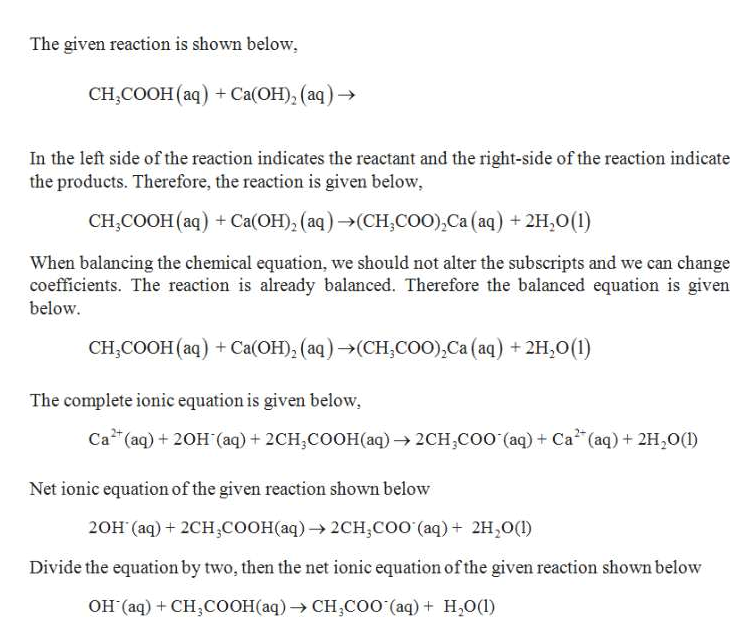# Complete the following acid-base reaction with balanced molecular, total ionic, and net ionic equations. Be sure to include states of matter in your answer. calcium hydroxide(aq) + acetic acid(aq)→Molecular:                             →Total Ionic: Reactants Products:→Net Ionic:                            →

Question
13 views

Complete the following acid-base reaction with balanced molecular, total ionic, and net ionic equations. Be sure to include states of matter in your answer.

calcium hydroxide(aq) + acetic acid(aq)→

 Molecular: → Total Ionic: Reactants Products: → Net Ionic: →
check_circle

Step 1

Balancing equation:

Balancing equation in which number of atoms for each element in the reaction and the total charge are same on both reactant side and the product side.

Steps in balancing the equation,...help_outlineImage TranscriptioncloseThe given reaction is shown below, CH,COОН (аq) + сСа(ОН), (аq) -> In the left side of the reaction indicates the reactant and the right-side of the reaction indicate the products. Therefore, the reaction is given below CH,COOH (aq) Ca(OH), (aq)(CH,coo),Ca (aq) +2H,0(1) When balancing the chemical equation, we should not alter the subscripts and we can change coefficients. The reaction is already balanced. Therefore the balanced equation is given below CH,COOH (aq) Ca(OH), (aq)(CH,coo),Ca (aq) +2H,0(1) The complete ionic equation is given below Ca (aq)20H (aq) + 2CH,COOH(aq)2CH,Coo (aq) Ca (aq)21H20() Net ionic equation of the given reaction shown below 20H (aq) 2CH,COOH(aq) 2CH,coo (aq) 2H,O() Divide the equation by two, then the net ionic equation ofthe given reaction shown below он (аq) + CH,CоОНаaq) -> сн,Соо (aq) + H,0() fullscreen

### Want to see the full answer?

See Solution

#### Want to see this answer and more?

Solutions are written by subject experts who are available 24/7. Questions are typically answered within 1 hour.*

See Solution
*Response times may vary by subject and question.
Tagged in

### General Chemistry